## How to round number to nearest 5/10/50/100 in Excel?

Sometimes, you may want to convert numbers to nearest 5/10/50/100 for calculation in Excel. This Tutorial provides some simple formulas to round numbers to nearest specific number, and also introduce the formulas to round numbers to next or last nearest one.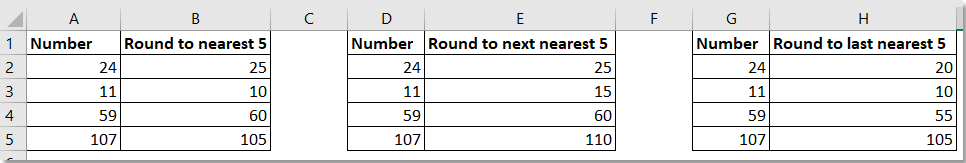Round numbers to nearest 5

####Round numbers to nearest 5

Round to nearest 5/10/50/100

 Round to nearest 5 =ROUND(A2/5,0)*5 Round to nearest 10 =ROUND(A2/10,0)*10 Round to nearest 50 =ROUND(A2/50,0)*50 Round to nearest 100 =ROUND(A2/100,0)*100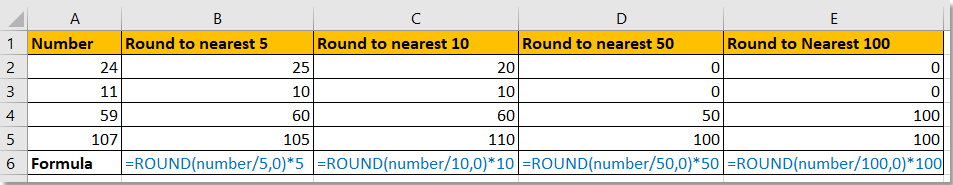Note:

You also can use this formula =MROUND(A2,5) to round numbers to nearest 5.

Round to next nearest 5/10/50/100

 Round to next nearest 5 =ROUNDUP(A2/5,0)*5 Round to next nearest 10 =ROUNDUP(A2/10,0)*10 Round to next nearest 50 =ROUNDUP(A2/50,0)*50 Round to next nearest 100 =ROUNDUP(A2/100,0)*100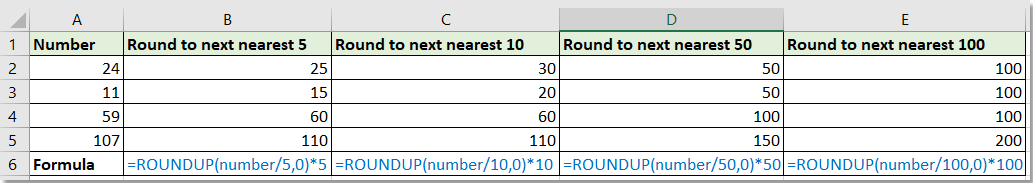Round to last nearest 5/10/50/100

 Round to last nearest 5 =ROUNDDOWN(A2/5,0)*5 Round to last nearest 10 =ROUNDDOWN(A2/10,0)*10 Round to last nearest 50 =ROUNDDOWN(A2/50,0)*50 Round to last nearest 100 =ROUNDDOWN(A2/100,0)*100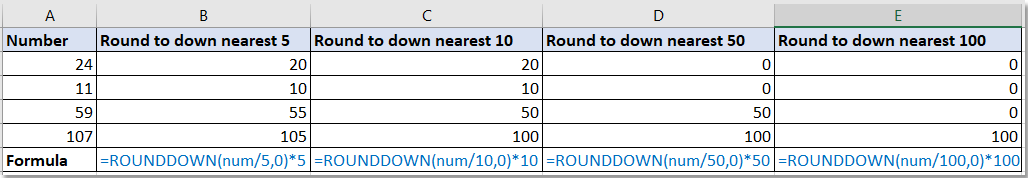#### Easily Round numbers up or down or to even in Excel without formula

As we known, there are some formulas can round numbers, but you do not want to use formula to round numbers, the Round utility of Kutools for Excel can do you a favor. Click for 30 days free trial!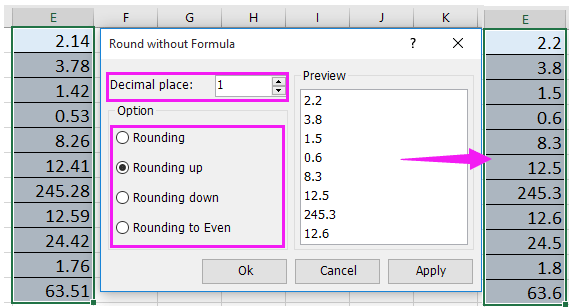Kutools for Excel: with more than 300 handy Excel add-ins, free to try with no limitation in 30 days.

### Best Office Productivity Tools

Supercharge Your Spreadsheets： Experience Efficiency Like Never Before with Kutools for Excel

 Popular Features: Find/Highlight/Identify Duplicates   |  Delete Blank Rows   |  Combine Columns or Cells without Losing Data   |   Round without Formula ... Super Lookup: Multiple Criteria VLookup  |   Multiple Value VLookup  |   VLookup Across Multiple Sheets   |   Fuzzy Lookup .... Advanced Drop-down List: Quickly Create Drop Down List   |  Dependent Drop Down List   |  Multi-select Drop Down List .... Column Manager: Add a Specific Number of Columns   |   Move Columns   |   Unhide Columns   |   Compare Columns to Select Same & Different Cells ... Featured Features: Grid Focus   |  Design View   |   Big Formula Bar   |  Workbook & Sheet Manager   |  Resource Library (Auto Text)   |  Date Picker   |  Combine Worksheets   |  Encrypt/Decrypt Cells   |  Send Emails by List   |  Super Filter   |   Special Filter (filter bold/italic/strikethrough...) ... Top 15 Toolset:  12 Text Tools (Add Text, Remove Characters, ...)   |   50+ Chart Types (Gantt Chart, ...)   |   40+ Practical Formulas (Calculate age based on birthday, ...)   |   19 Insertion Tools (Insert QR Code, Insert Picture from Path, ...)   |   12 Conversion Tools (Numbers to Words, Currency Conversion, ...)   |   7 Merge & Split Tools (Advanced Combine Rows, Split Cells, ...)   |   Many More...

Kutools for Excel boasts over 300 features, ensuring that what you need is just a click away...

Supports Office/Excel 2007-2021 & newer, including 365   |   Available in 44 languages   |   Enjoy a full-featured 30-day free trial.#### Office Tab Brings Tabbed interface to Office, and Make Your Work Much Easier

• Enable tabbed editing and reading in Word, Excel, PowerPoint, Publisher, Access, Visio and Project.
• Open and create multiple documents in new tabs of the same window, rather than in new windows.
• Increases your productivity by 50%, and reduces hundreds of mouse clicks for you every day!No ratings yet. Be the first to rate!
This comment was minimized by the moderator on the site
may i know how to set a formula which include 2 criteria, 1/2 remain 1/2 and every 3/4 round up to 1, e.g. 3/4->1, 1-3/4->2, 2-3/4->3
This comment was minimized by the moderator on the site
I want to round up in the same columm,

Example : 1201 to be 1250
This comment was minimized by the moderator on the site
I want to round up or down decimal places in the same columm,

Example : 12.49 to be 12 and 12.50 to be 13
This comment was minimized by the moderator on the site
I want to generate an IF formula that will help me round up or down decimals in the same column

example :

Cell A1 ----- 6.13 want it to round up to 6.49
Cell A2 ----- 6.74 want it to round up to 6.79
Cell A3 ----- 6.84 want it to round down to 6.79
This comment was minimized by the moderator on the site
Sorry, Jose, I do not understand your round up and down rule.
This comment was minimized by the moderator on the site
I want to generate an IF formula that will help me round up or down decimals in the same column

example :

Cell A1 ----- 6.13 want it to round up to 6.49
Cell A2 ----- 6.74 want it to round up to 6.79
Cell A3 ----- 6.84 want it to round down to 6.79
This comment was minimized by the moderator on the site
HOW TO ROUNDUP 2.5 =5 AND 7.8=10
This comment was minimized by the moderator on the site
Use this formula =mround("cell address where 2.5 or 7.8 is written in the excel sheet",5) i.e. if 2.5 is written in cell A1 then =mround(a1,5) and if 7.8 is written in cell B1 then =mround(b1,5)
This comment was minimized by the moderator on the site
Sorry I have no idea.
This comment was minimized by the moderator on the site
i need formula that will write 95000 as 90000 or 87000 as 80000, if figure 89000 then it should be 90000.
Means only 9 or above then should mround to 10 otherwise not
This comment was minimized by the moderator on the site
You can go to our forum to submit your question, someone may answer you. https://www.extendoffice.com/forum.html
This comment was minimized by the moderator on the site
HOW TO ROUND 50.49 =50 AND 50.50=60 AND 90.49=90 AND 90.50=100
This comment was minimized by the moderator on the site
Hello, you just need this formula =ROUND(reference, 0).
This comment was minimized by the moderator on the site
well articulated article, worked for me thanks
This comment was minimized by the moderator on the site
Can I use this in a formula? For example, I want the formula =(C118*D118)+50, but I want the answer to round up to the nearest five. So if C118 is 216, and D118 is 13, I want 2808 + 50 to round up to 2860. I'd like to to it all in the same column rather than adding a column. Thanks!
This comment was minimized by the moderator on the site
use this formula =ceiling((c118*d118)+50,10)
This comment was minimized by the moderator on the site
Is there a formula that you can set so that decimals are rounded up to the nearest .05? i.e. 3.13 = 3.15 OR 2.11 = 2.15
This comment was minimized by the moderator on the site
=ROUNDUP(A2/0.05,0)*0.05
This comment was minimized by the moderator on the site
Can I roundup to the nearest even number?
This comment was minimized by the moderator on the site
Maybe you can try =EVEN(A1).
This comment was minimized by the moderator on the site
How to put 2 formulas in 1 cell. 1st formula is the quotient of 2 cells, and this result should be rounded up to the nearest 10.
This comment was minimized by the moderator on the site
Hi, supposing A1 and B1 is the two cells that you want to get their quotient, and to roundup the quotient to nearest 10, you can use the formula as this: =ROUNDUP(A1/B1/10,0)*10.
There are no comments posted here yet